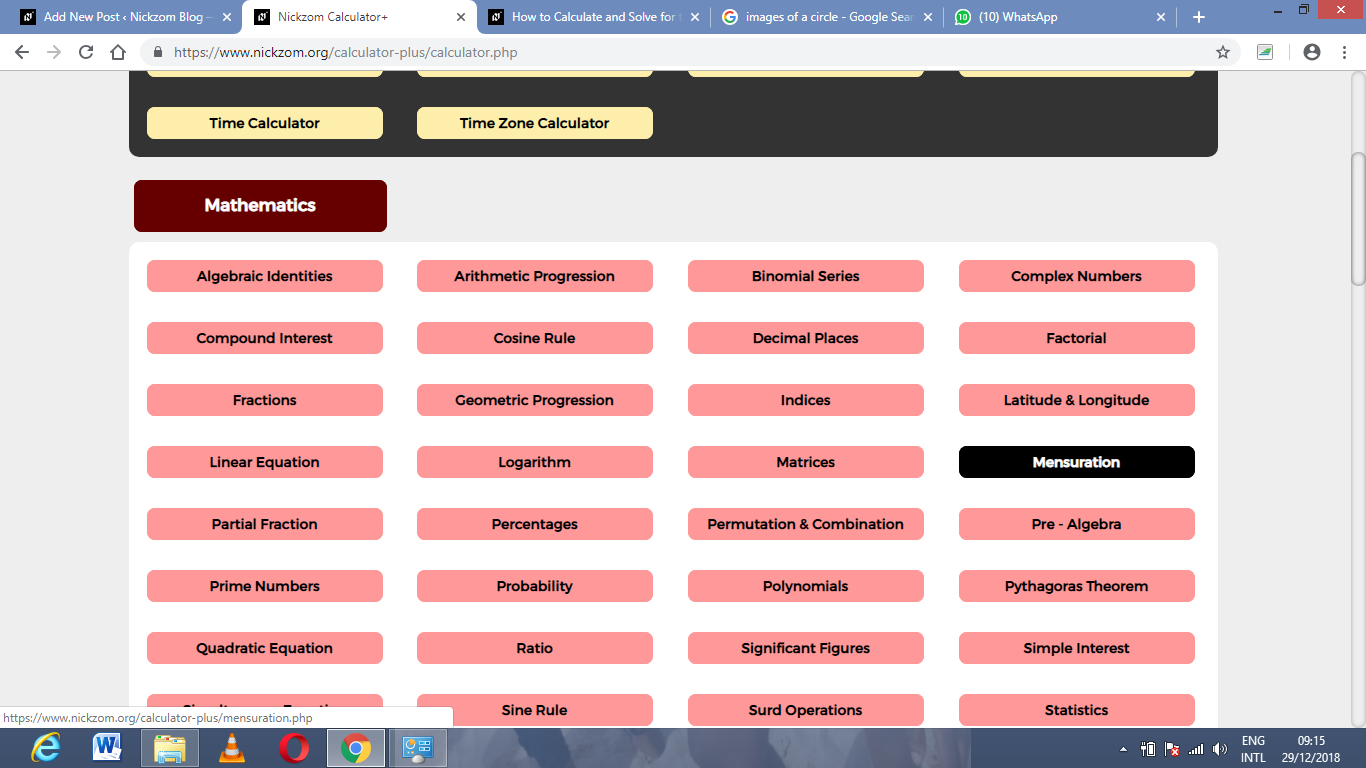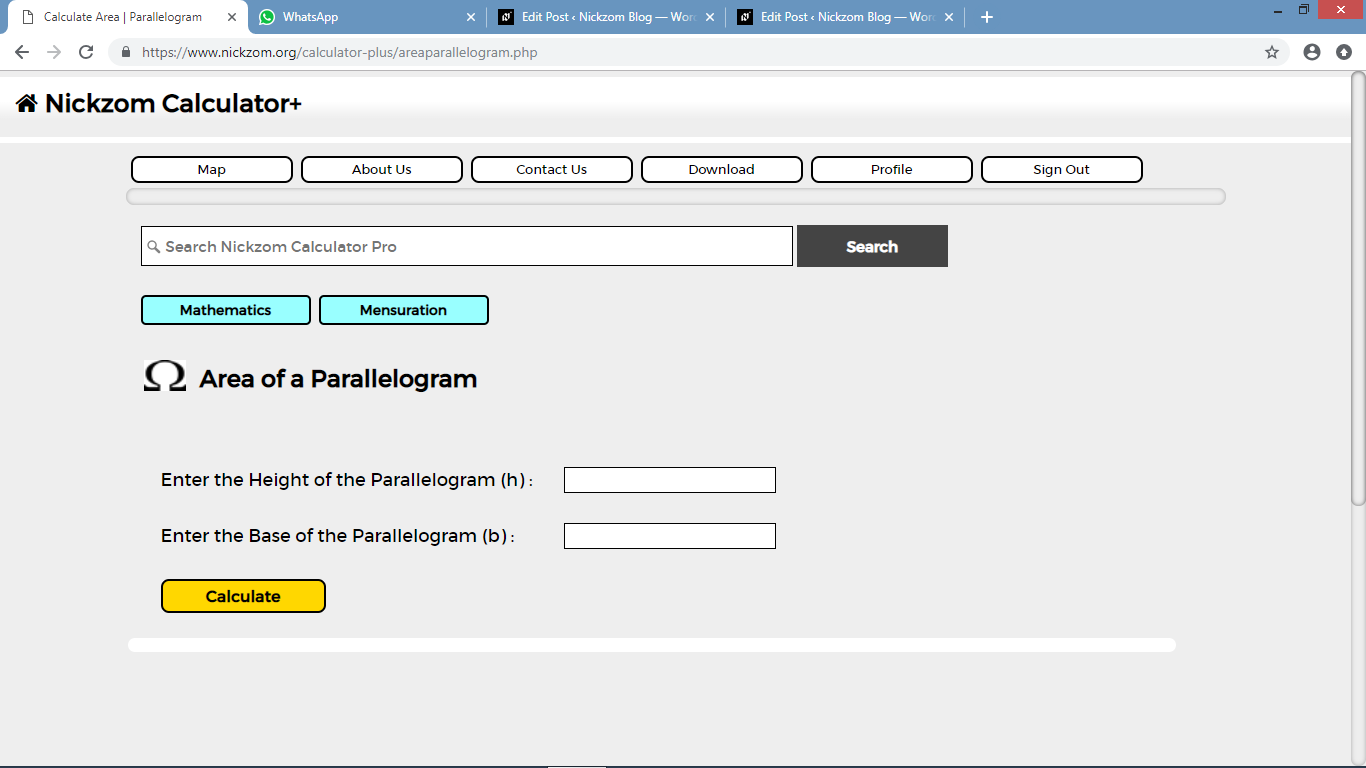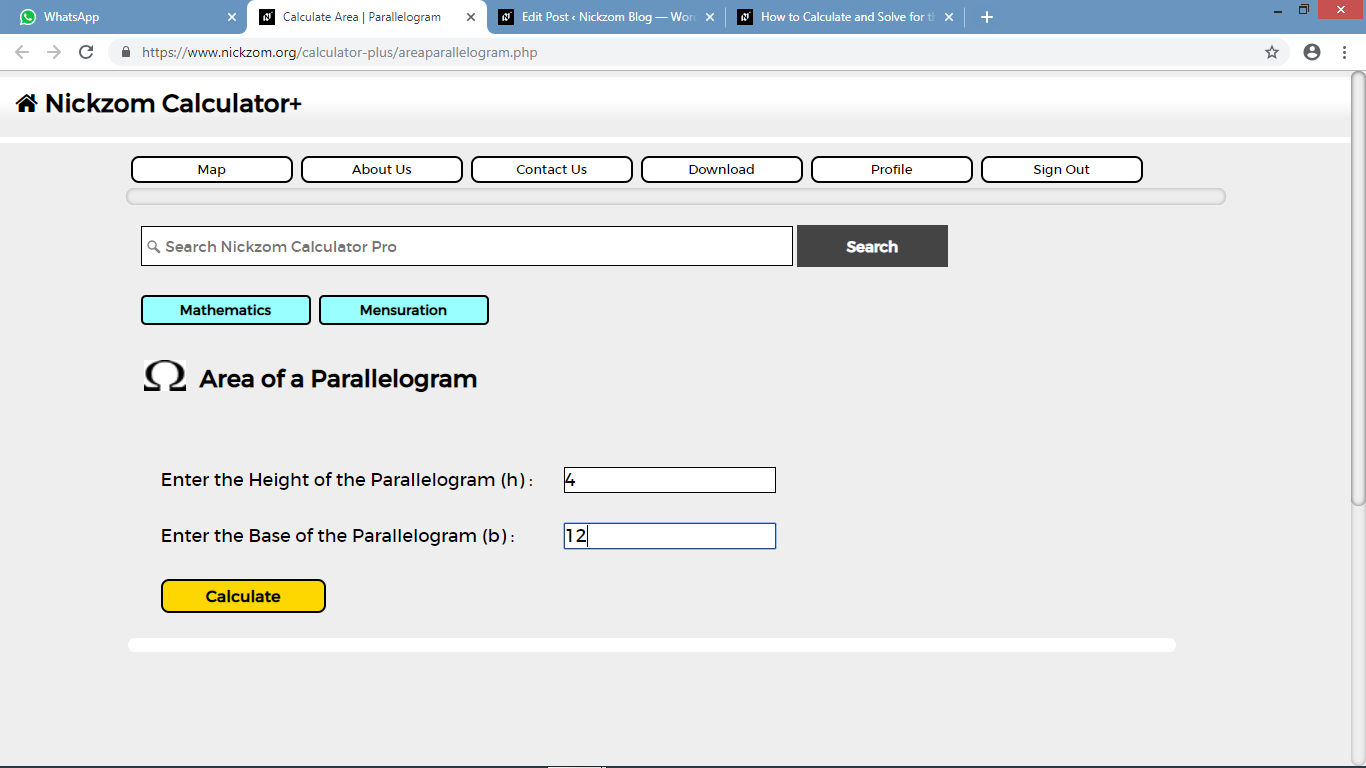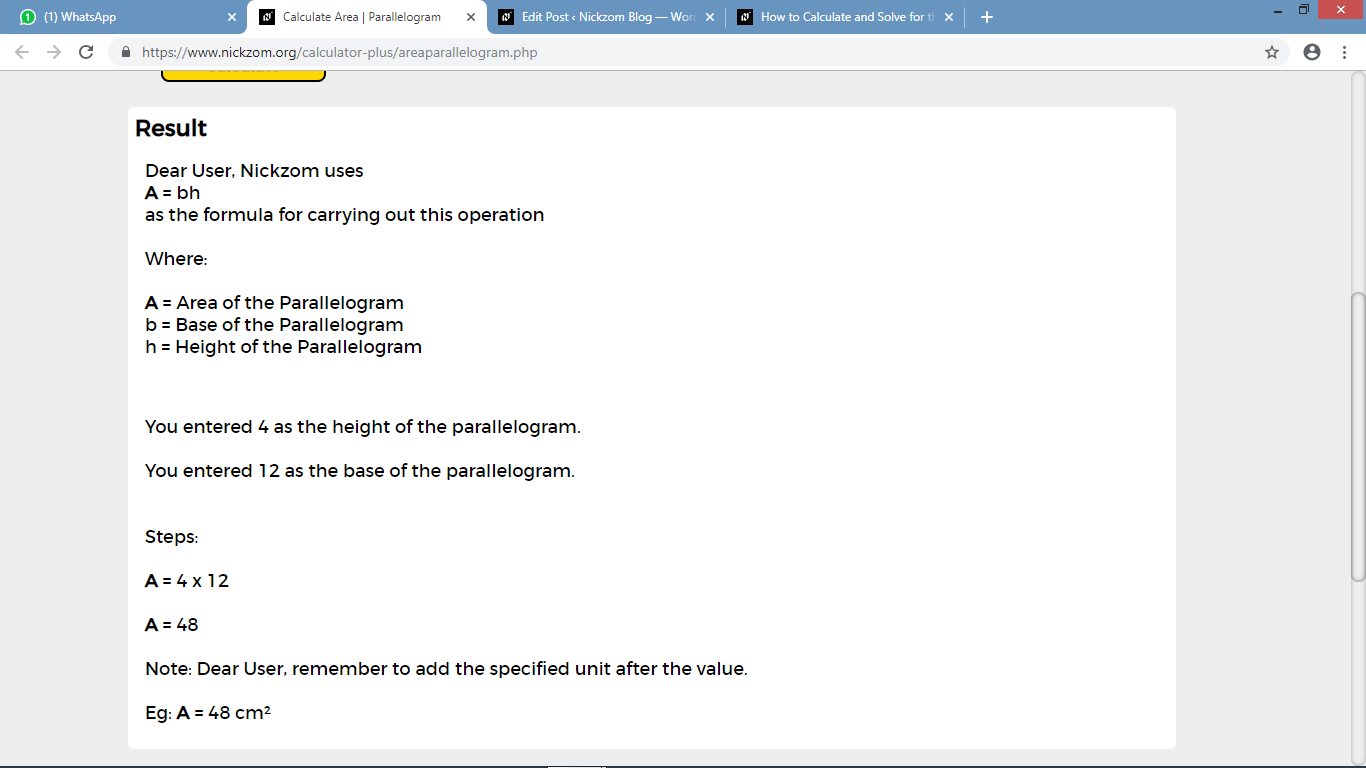# How to Calculate and Solve for the Area and Length of a Parallelogram | The Calculator EncyclopediaThe image above represents a parallelogram.
To compute the area of a parallelogram requires two essential parameters which are the base and height of the parallelogram.

The formula for computing the area of a parallelogram is:

A = b x h

Where:
A = Area of a parallelogram
b = base of the parallelogram
h = height of the parallelogram

Let’s solve an example
Find the area of a parallelogram with a base of 12 cm and a height of 4 cm.

This implies that:
b = base of the parallelogram = 12
h = height of the parallelogram = 4

A = b x h
A = 12 x 4
A = 48

Therefore, the area of the parallelogram is 48 cm2.

Calculating the base of a parallelogram when Area and Height is Given

The formula is b = A / h

Where;
A = Area of a parallelogram
b = base of the parallelogram
h = height of the parallelogram

Let’s solve an example:
Find the base of a parallelogram with an area of 40 cm² and a height of 8 cm

This implies that;
A = Area of the parallelogram = 40 cm²
h = height of the parallelogram = 8 cm

b = A / h
b = 40 / 8
b = 5

Therefore, the base of the parallelogram is 5 cm.

Calculating the height of a parallelogram when Area and Base is Given

The formula is h = A / b

Where;
A = Area of a parallelogram
b = base of the parallelogram
h = height of the parallelogram

Let’s solve an example:
Find the height of a parallelogram with an area of 60 cm² and a base of 6 cm

This implies that;
A = Area of the parallelogram = 60 cm²
b = base of the parallelogram = 6 cm

h = A / b
h = 60 / 6
h = 10

Therefore, the height of the parallelogram is 10 cm.

Nickzom Calculator – The Calculator Encyclopedia is capable of calculating the area of a parallelogram.

To get the answer and workings of the perimeter of a square using the Nickzom Calculator – The Calculator Encyclopedia. First, you need to obtain the app.

You can get this app via any of these means:

To get access to the professional version via web, you need to register and subscribe for NGN 1,500 per annum to have utter access to all functionalities.
You can also try the demo version via https://www.nickzom.org/calculator

Once, you have obtained the calculator encyclopedia app, proceed to the Calculator Map, then click on Mensuration under the Mathematics section.Now, click on Area of a Parallelogram under MensurationThe screenshot below displays the page or activity to enter your values, to get the answer for the area of a parallelogram according to the respective parameters which are the base and height of the parallelogram.Now, enter the values appropriately and accordingly for the parameters as required by the example above where the base of the parallelogram is 12 cm and the height of the parallelogram is 4 cm.Finally, click on the Calculate buttonAs you can see from the screenshot above, Nickzom Calculator – The Calculator Encyclopedia solves for the area of a parallelogram and presents the formula, workings and steps too.